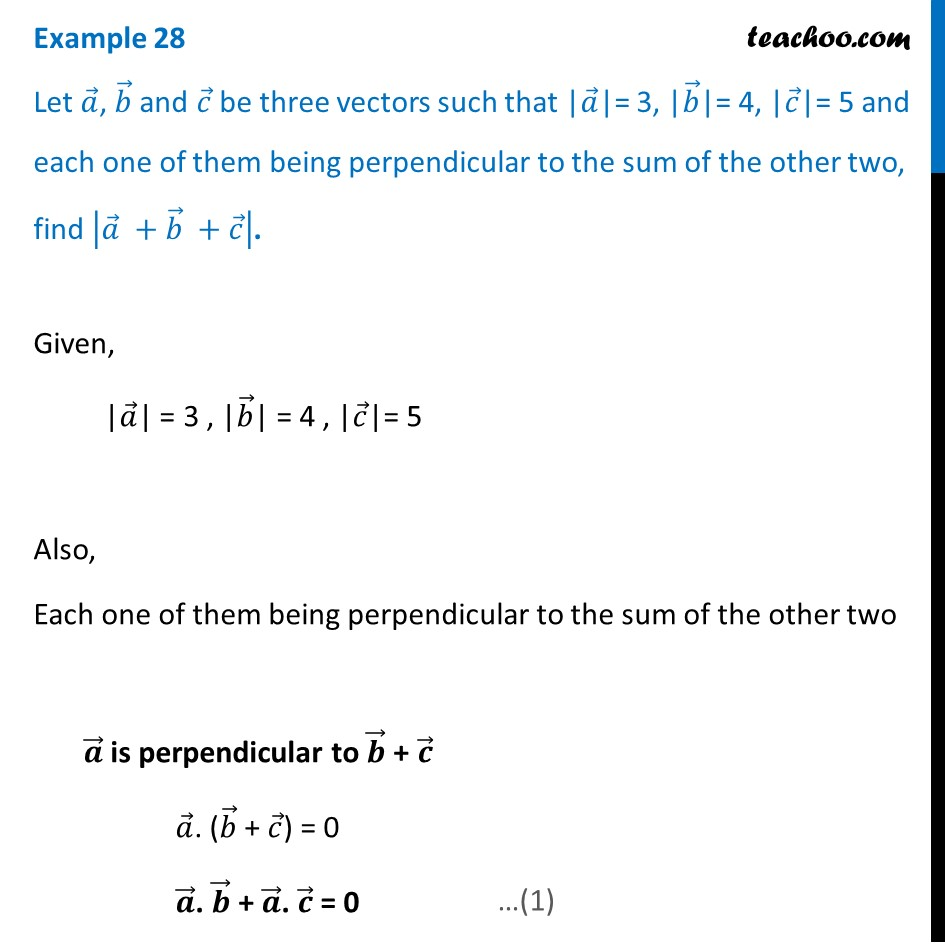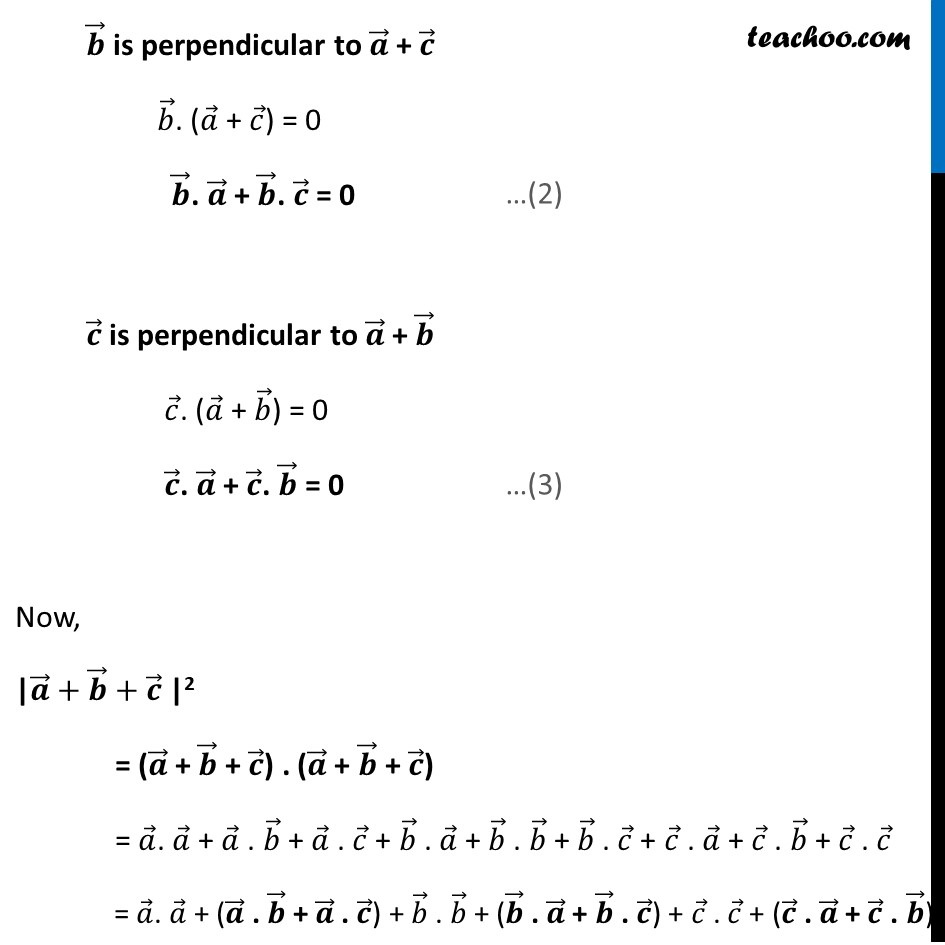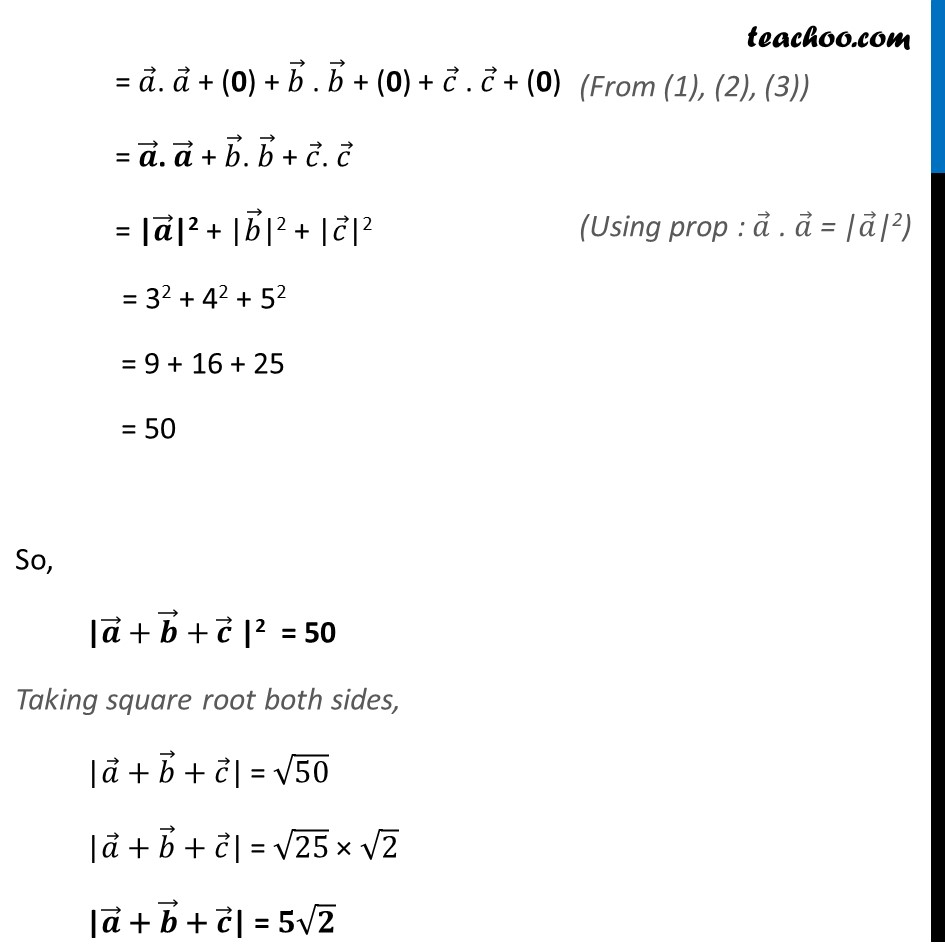Scalar product - Solving

Chapter 10 Class 12 Vector Algebra
Concept wiseLearn in your speed, with individual attention - Teachoo Maths 1-on-1 Class

### Transcript

Example 28 Let 𝑎 ⃗, 𝑏 ⃗ and 𝑐 ⃗ be three vectors such that |𝑎 ⃗|= 3, |𝑏 ⃗|= 4, |𝑐 ⃗|= 5 and each one of them being perpendicular to the sum of the other two, find |𝑎 ⃗" + " 𝑏 ⃗" + " 𝑐 ⃗ |.Given, |𝑎 ⃗| = 3 , |𝑏 ⃗| = 4 , |𝑐 ⃗|= 5 Also, Each one of them being perpendicular to the sum of the other two 𝒂 ⃗ is perpendicular to 𝒃 ⃗ + 𝒄 ⃗ 𝑎 ⃗. (𝑏 ⃗ + 𝑐 ⃗) = 0 𝒂 ⃗. 𝒃 ⃗ + 𝒂 ⃗. 𝒄 ⃗ = 0 𝒃 ⃗ is perpendicular to 𝒂 ⃗ + 𝒄 ⃗ 𝑏 ⃗. (𝑎 ⃗ + 𝑐 ⃗) = 0 𝒃 ⃗. 𝒂 ⃗ + 𝒃 ⃗. 𝒄 ⃗ = 0 𝒄 ⃗ is perpendicular to 𝒂 ⃗ + 𝒃 ⃗ 𝑐 ⃗. (𝑎 ⃗ + 𝑏 ⃗) = 0 𝒄 ⃗. 𝒂 ⃗ + 𝒄 ⃗. 𝒃 ⃗ = 0 Now, |𝒂 ⃗+𝒃 ⃗+𝒄 ⃗ |2 = (𝒂 ⃗ + 𝒃 ⃗ + 𝒄 ⃗) . (𝒂 ⃗ + 𝒃 ⃗ + 𝒄 ⃗) = 𝑎 ⃗. 𝑎 ⃗ + 𝑎 ⃗ . 𝑏 ⃗ + 𝑎 ⃗ . 𝑐 ⃗ + 𝑏 ⃗ . 𝑎 ⃗ + 𝑏 ⃗ . 𝑏 ⃗ + 𝑏 ⃗ . 𝑐 ⃗ + 𝑐 ⃗ . 𝑎 ⃗ + 𝑐 ⃗ . 𝑏 ⃗ + 𝑐 ⃗ . 𝑐 ⃗ = 𝑎 ⃗. 𝑎 ⃗ + (𝒂 ⃗ . 𝒃 ⃗ + 𝒂 ⃗ . 𝒄 ⃗) + 𝑏 ⃗ . 𝑏 ⃗ + (𝒃 ⃗ . 𝒂 ⃗ + 𝒃 ⃗ . 𝒄 ⃗) + 𝑐 ⃗ . 𝑐 ⃗ + (𝒄 ⃗ . 𝒂 ⃗ + 𝒄 ⃗ . 𝒃 ⃗ = 𝑎 ⃗. 𝑎 ⃗ + (0) + 𝑏 ⃗ . 𝑏 ⃗ + (0) + 𝑐 ⃗ . 𝑐 ⃗ + (0) = 𝒂 ⃗. 𝒂 ⃗ + 𝑏 ⃗. 𝑏 ⃗ + 𝑐 ⃗. 𝑐 ⃗ = "|" 𝒂 ⃗"|"2 + "|" 𝑏 ⃗"|"2 + "|" 𝑐 ⃗"|"2 = 32 + 42 + 52 = 9 + 16 + 25 = 50 So, |𝒂 ⃗+𝒃 ⃗+𝒄 ⃗ |2 = 50 Taking square root both sides, "|" 𝑎 ⃗ "+ " 𝑏 ⃗" + " 𝑐 ⃗"|" = √50 "|" 𝑎 ⃗ "+ " 𝑏 ⃗ "+ " 𝑐 ⃗"|" = √25 × √2 "|" 𝒂 ⃗ "+ " 𝒃 ⃗ "+ " 𝒄 ⃗"|" = 𝟓√𝟐 = 𝑎 ⃗. 𝑎 ⃗ + (0) + 𝑏 ⃗ . 𝑏 ⃗ + (0) + 𝑐 ⃗ . 𝑐 ⃗ + (0) = 𝒂 ⃗. 𝒂 ⃗ + 𝑏 ⃗. 𝑏 ⃗ + 𝑐 ⃗. 𝑐 ⃗ = "|" 𝒂 ⃗"|"2 + "|" 𝑏 ⃗"|"2 + "|" 𝑐 ⃗"|"2 = 32 + 42 + 52 = 9 + 16 + 25 = 50 So, |𝒂 ⃗+𝒃 ⃗+𝒄 ⃗ |2 = 50 Taking square root both sides, "|" 𝑎 ⃗ "+ " 𝑏 ⃗" + " 𝑐 ⃗"|" = √50 "|" 𝑎 ⃗ "+ " 𝑏 ⃗ "+ " 𝑐 ⃗"|" = √25 × √2 "|" 𝒂 ⃗ "+ " 𝒃 ⃗ "+ " 𝒄 ⃗"|" = 𝟓√𝟐